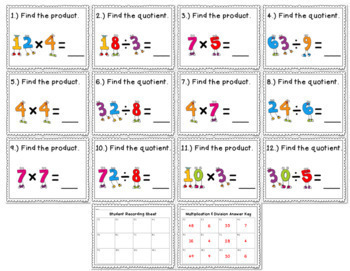# FREE Digital Task Cards: Multiplication & DivisionSubject
Resource Type
File Type

(12 pages)
StandardsMade for Google Drive™
• Product Description
• Standards

Looking for Google Classroom activities to make math more engaging? These FREE digital task cards are paperless and require no prep! All you need is a device, a free Google account and access to the internet. Students type their answers directly on the slides in the provided text boxes. An optional printable recording sheet is included if you’d like your students to turn in a paper copy.

Be sure to check out my other digital math task cards below.

(Each set includes a self-grading quiz!):

Operations & Algebraic Thinking:

Word Problems (Sports Themed)

Word Problems (School Themed)

Properties of Multiplication

Factors & Multiples

Continuing Number Patterns & Skip Counting

Ways to Show Multiplication

Number & Operations in Base 10:

Place Value: Rounding, Comparing, Ordering, Number Forms

Comparing & Ordering Multi-digit Numbers

Rounding

Multiplying by 1 and 2-digit Numbers

Long Division

Number & Operations: Fractions:

Fractions: Identifying, Ordering, Comparing, Equivalence

Fractions on a Number Line

Simplifying Fractions

Multiplying Fractions by Whole Numbers

Mixed Numbers & Improper Fractions

Decimals: Identifying, Comparing, Ordering

Measurement & Data:

Measurement Conversions

Measuring Angles with a Protractor

Measuring to the Half & Quarter Inch (With Line Plots)

Line Plots

Telling Time to the Minute

Elapsed Time

Geometry:

Geometry: Lines, Angles, Polygons, Area/Perimeter

***All of the sets above are also part of a DISCOUNTED MEGA BUNDLE***

Interpret a multiplication equation as a comparison, e.g., interpret 35 = 5 × 7 as a statement that 35 is 5 times as many as 7 and 7 times as many as 5. Represent verbal statements of multiplicative comparisons as multiplication equations.
Fluently multiply and divide within 100, using strategies such as the relationship between multiplication and division (e.g., knowing that 8 × 5 = 40, one knows 40 ÷ 5 = 8) or properties of operations. By the end of Grade 3, know from memory all products of two one-digit numbers.
Determine the unknown whole number in a multiplication or division equation relating three whole numbers. For example, determine the unknown number that makes the equation true in each of the equations 8 × ? = 48, 5 = __ ÷ 3, 6 × 6 = ?.
Total Pages
12 pages
Included
Teaching Duration
N/A
Report this Resource to TpT
Reported resources will be reviewed by our team. Report this resource to let us know if this resource violates TpT’s content guidelines.